# RD Sharma Solutions for Class 10 Maths Chapter 11 Constructions

Grabbing the easy marks in an examination takes a little a bit of awareness of what and how to present an answer. When you are in the crucial stages of examination you just can’t miss those easy ones to qualify the examination. That’s why our expert team at BYJU’S has come up with the RD Sharma Solutions for making students know the correct procedure of attempting and answer the question of mathematics. This is definitely a must for all students who prepare in the last minute and can also be used of quick revision of formulae and methods.

## Download the PDF of RD Sharma For Class 10 Maths Chapter 11 Constructions here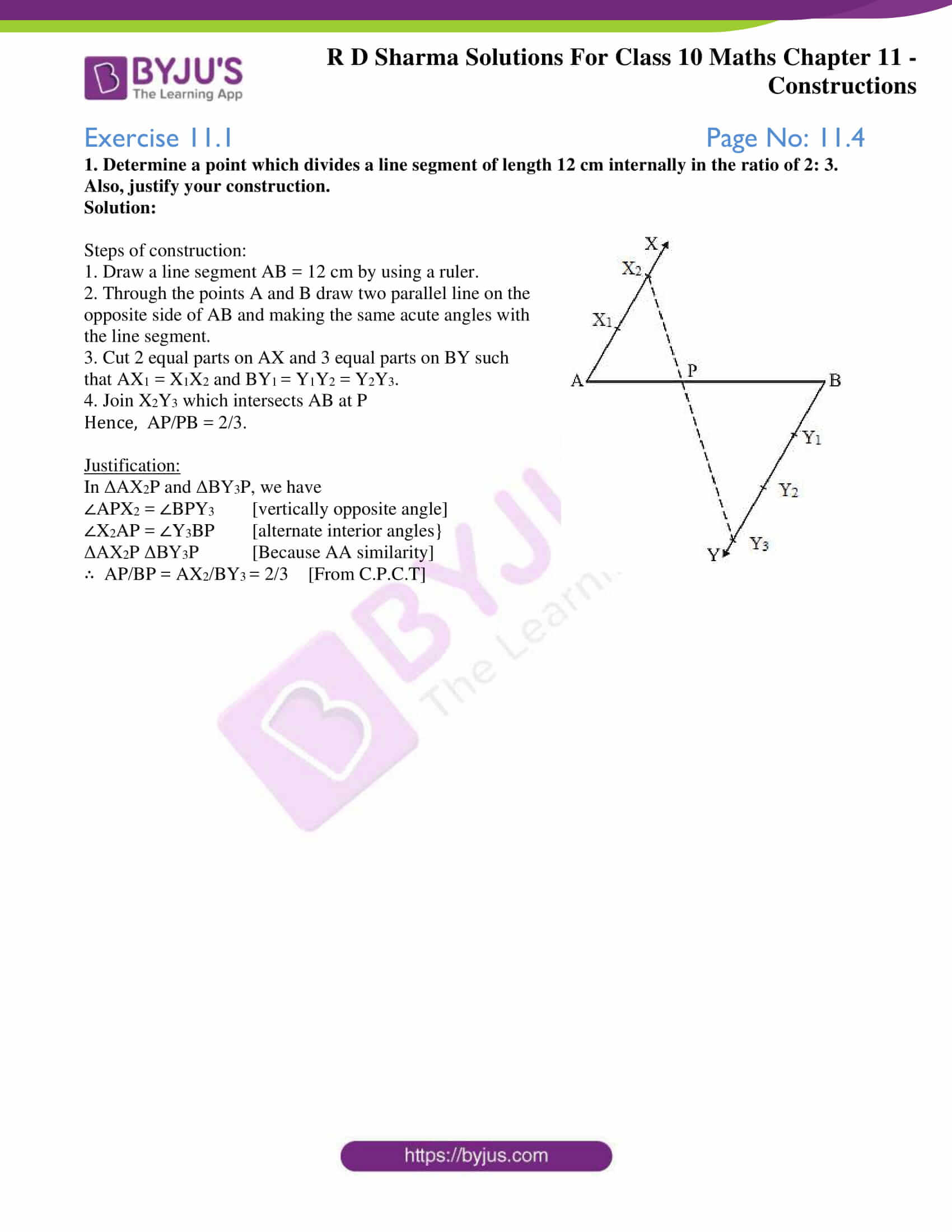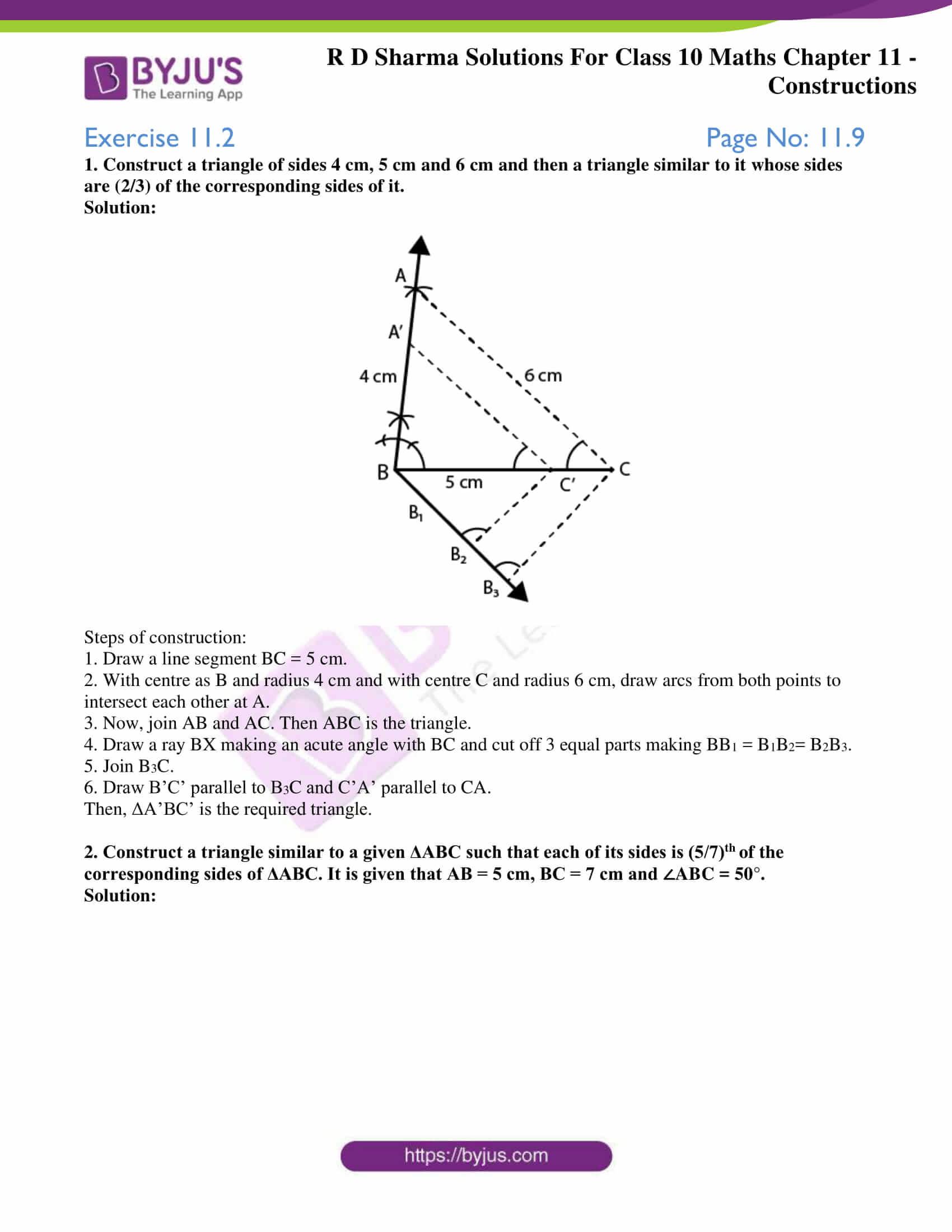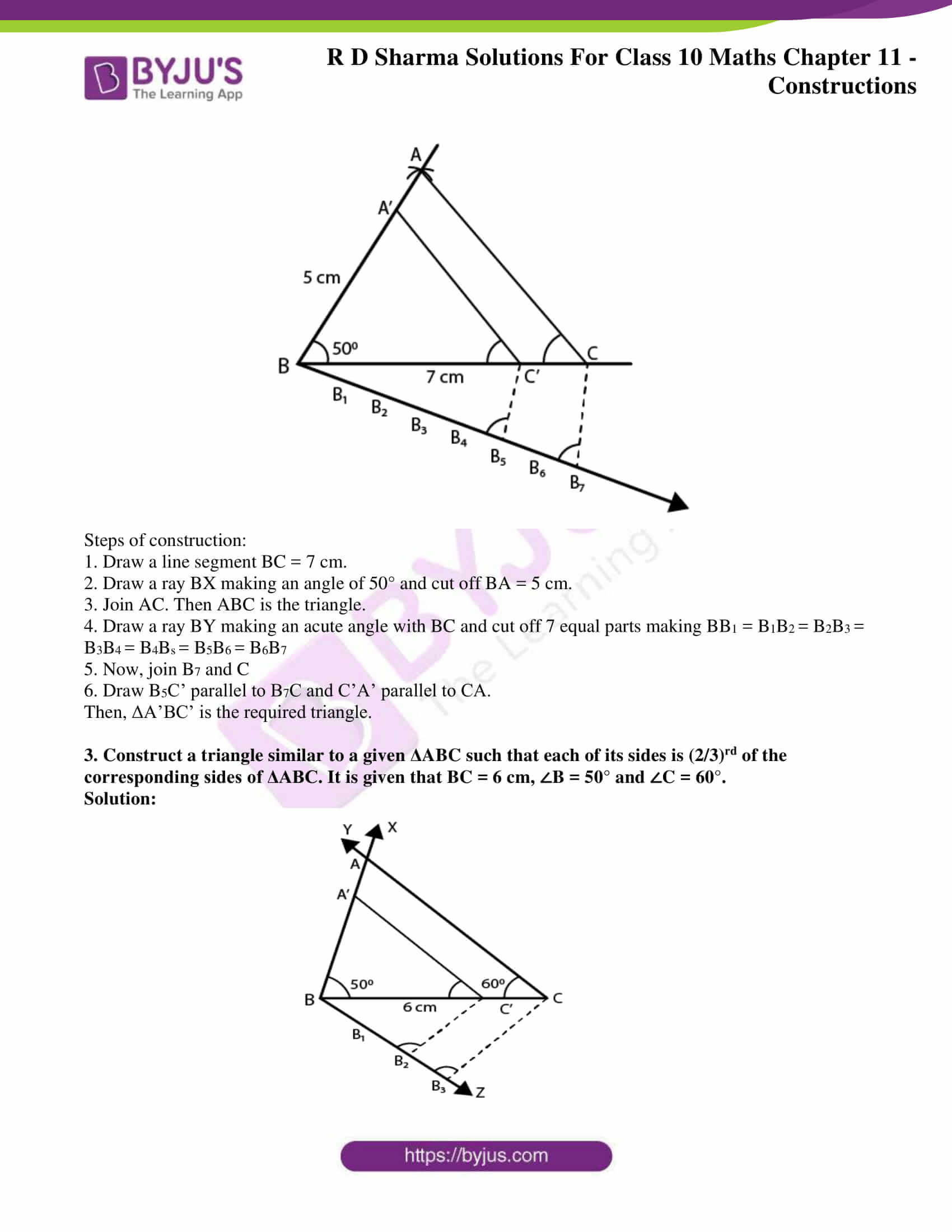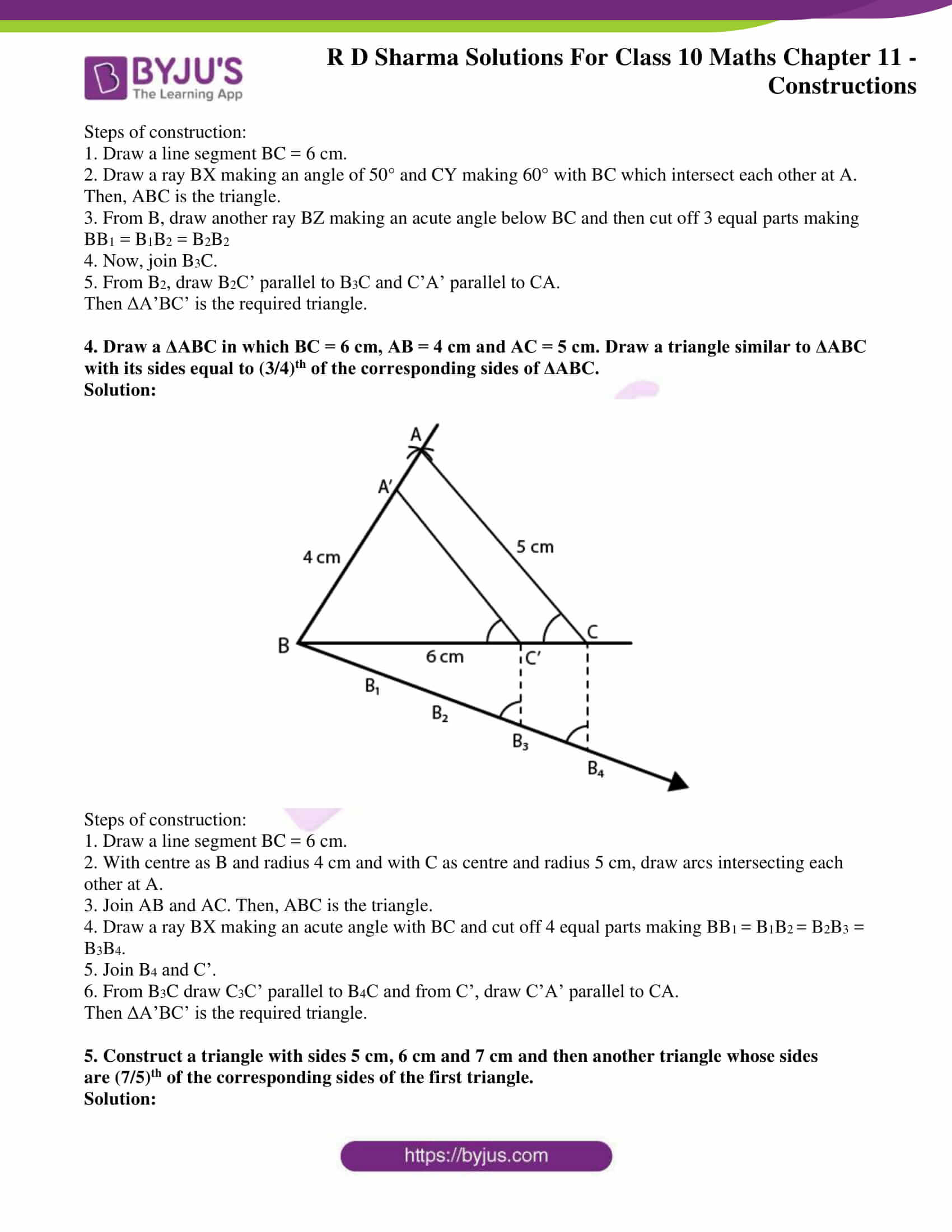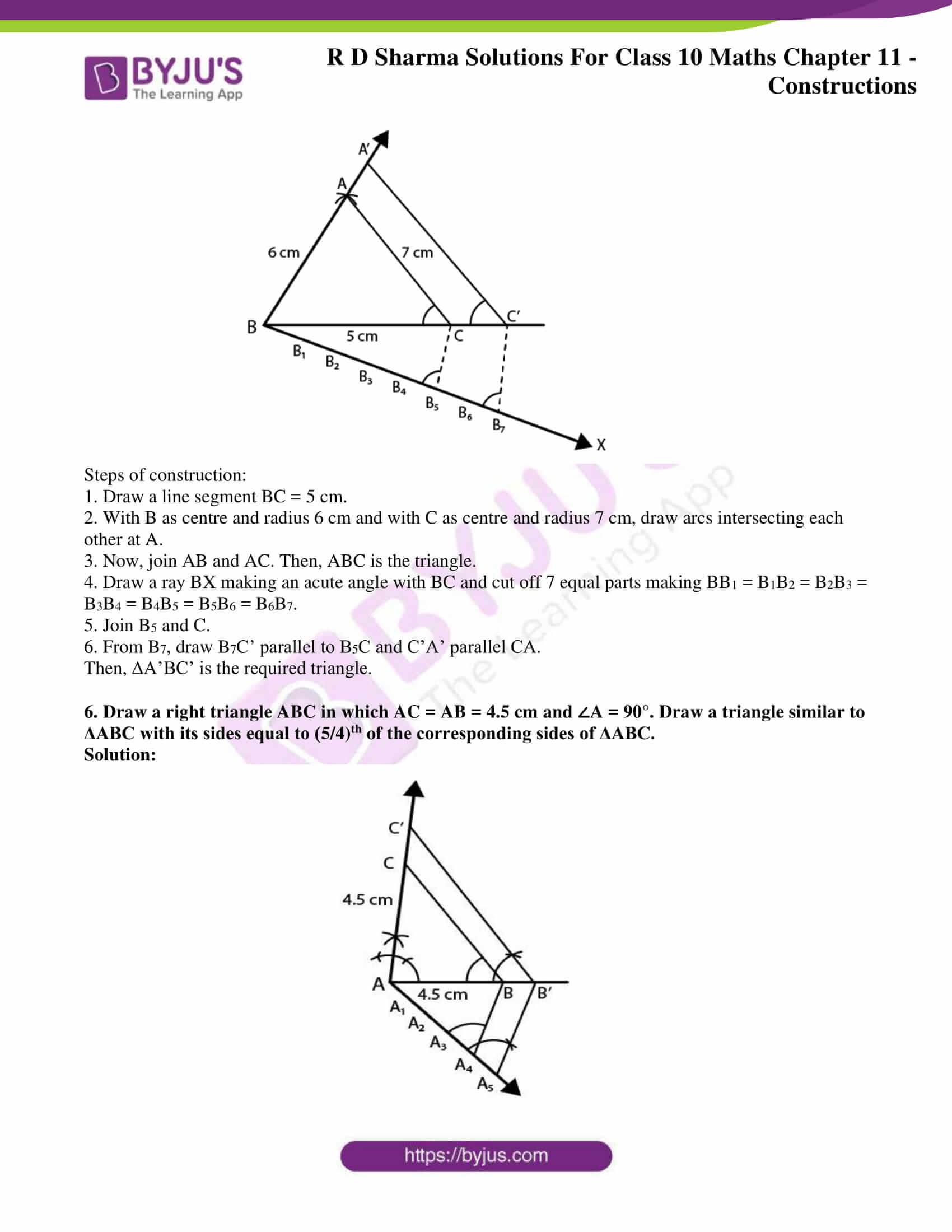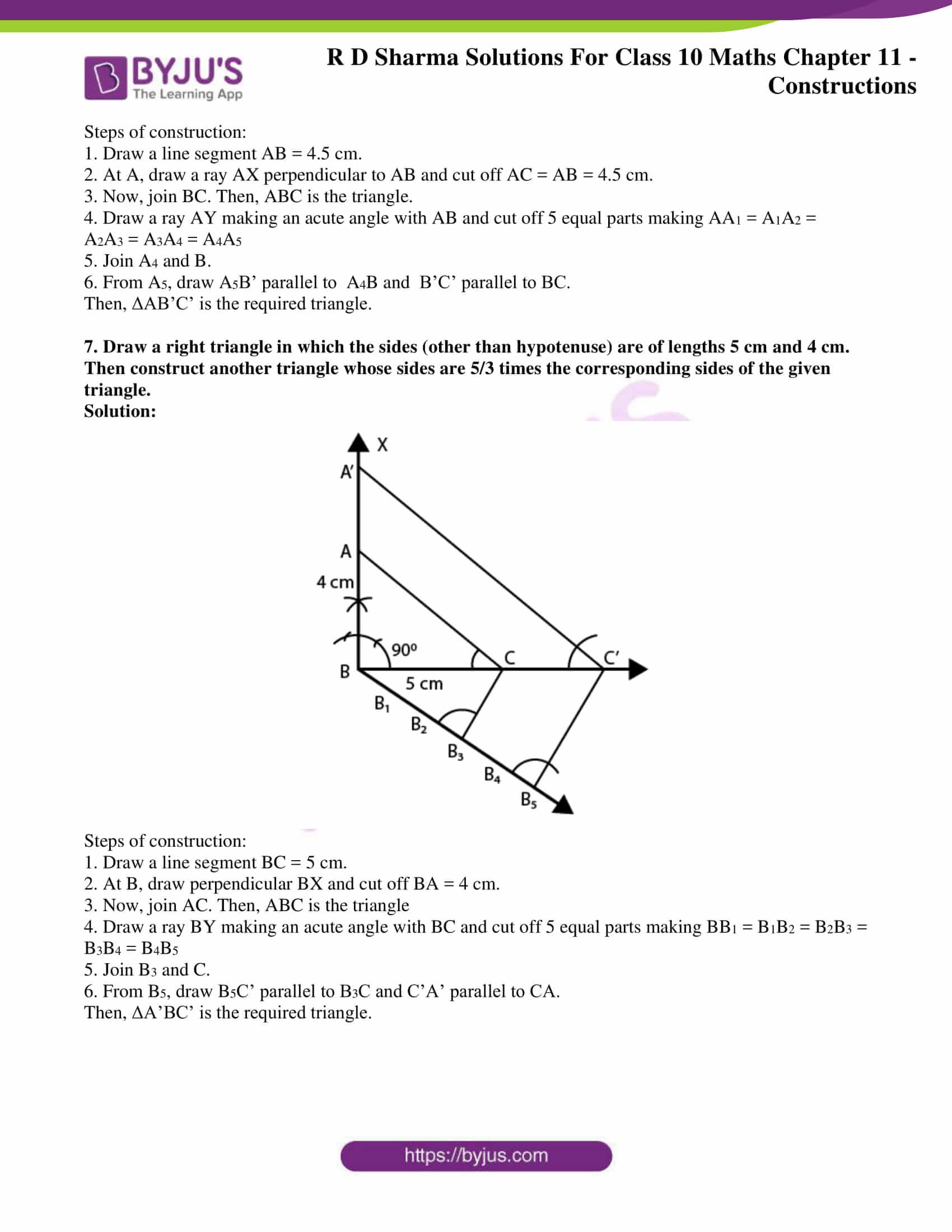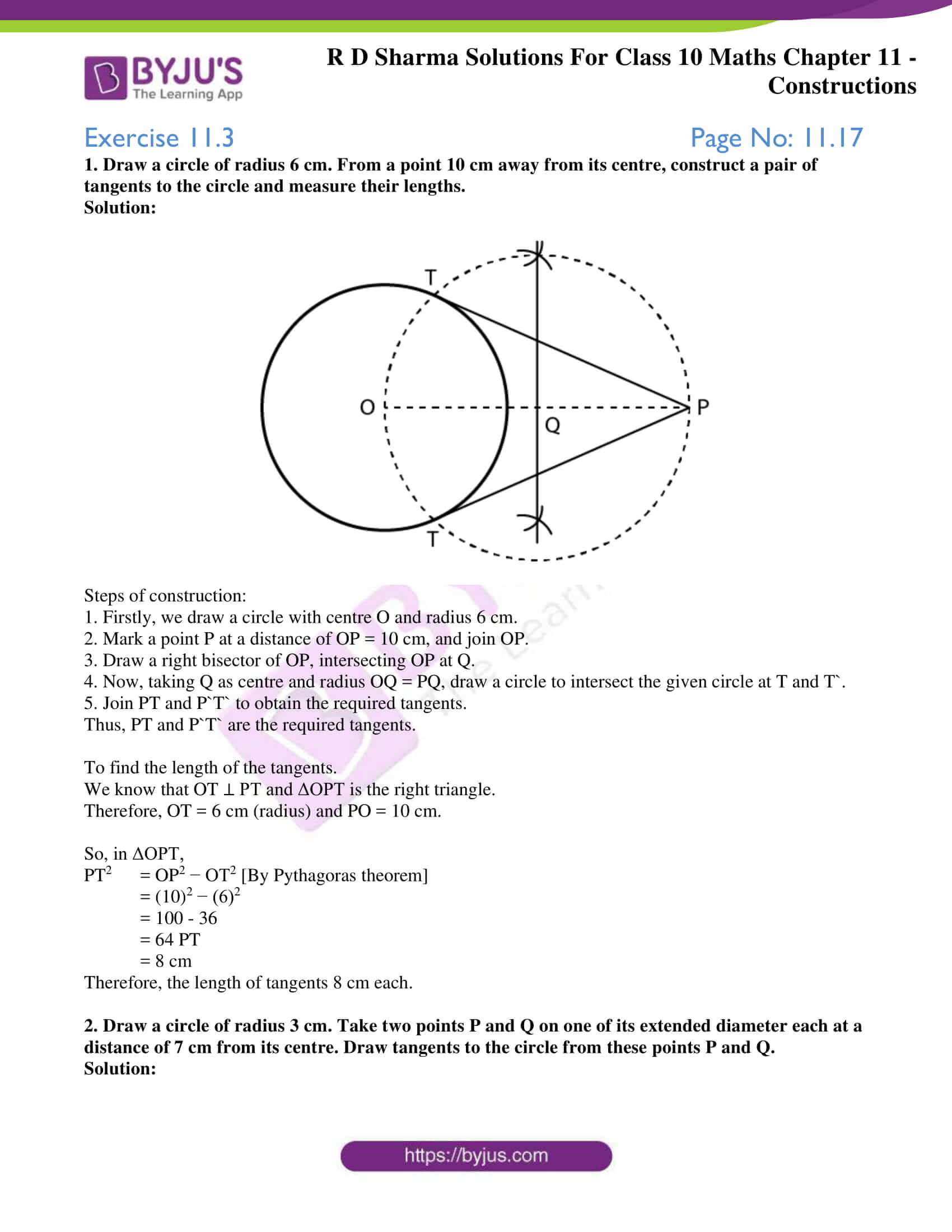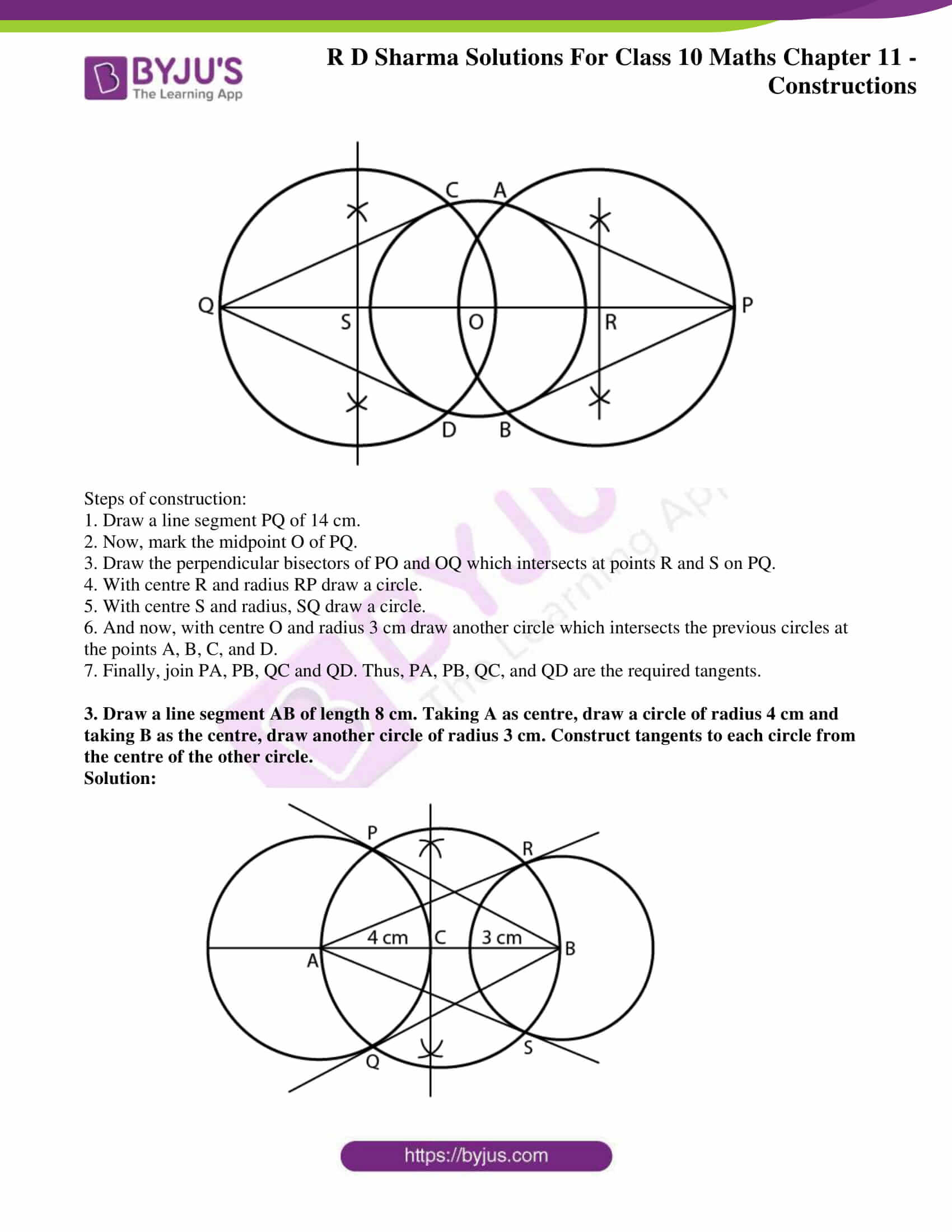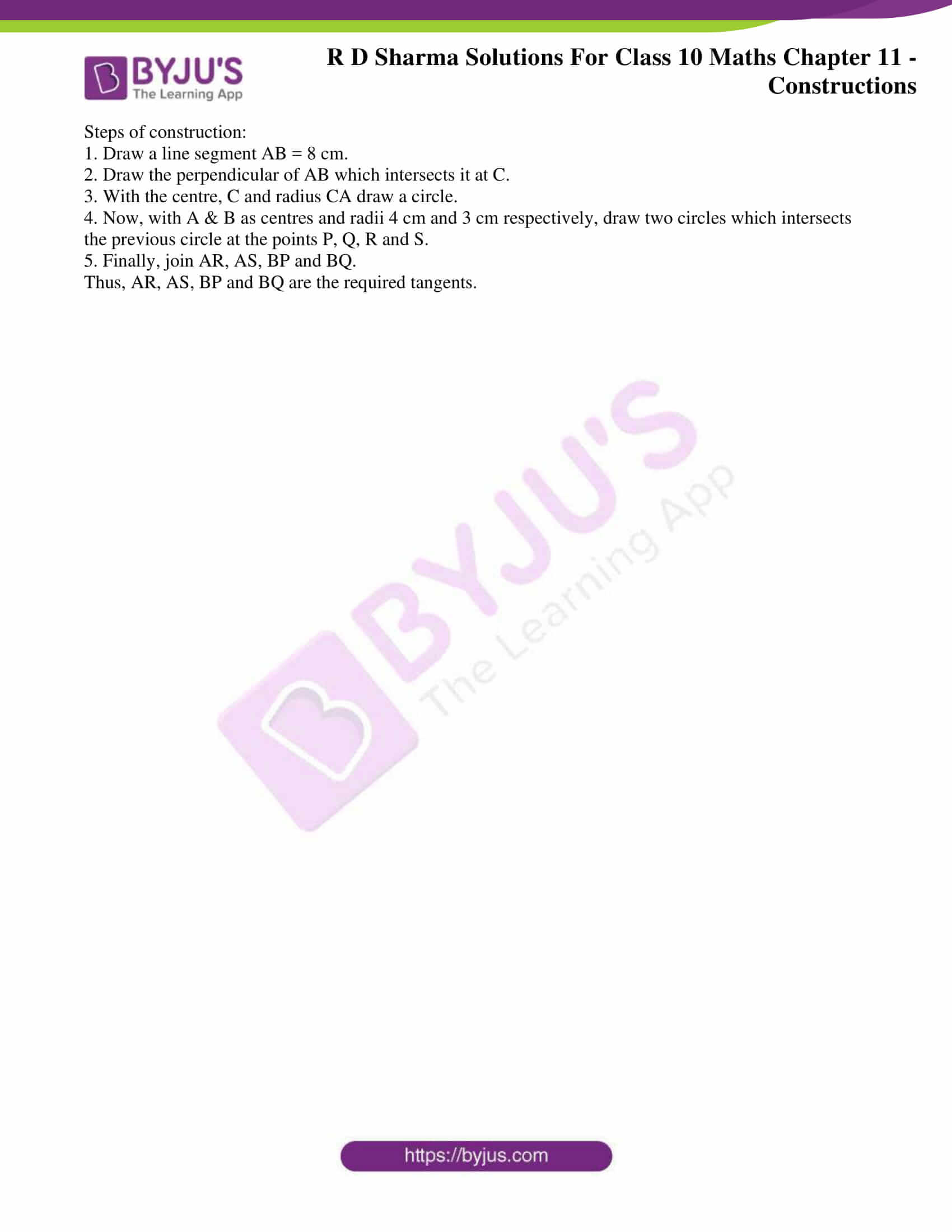Chapter – 11 Constructions has three exercises and you can check the RD Sharma Solutions for Class 10 to educate yourself with the right steps and presentation of these problems. Constructions basically in the continuation from the previous class and using that knowledge various other concepts of geometry like,

• Division of a line segment
• Construction of a triangle similar to a given triangle
• Construction of Tangents to a Circle

are constructed along with their justifications is learnt in this chapter.

### Access the RD Sharma Solutions For Class 10 Chapter 11 – Constructions

Exercise 11.1 Page No: 11.4

1. Determine a point which divides a line segment of length 12 cm internally in the ratio of 2: 3. Also, justify your construction.

Solution: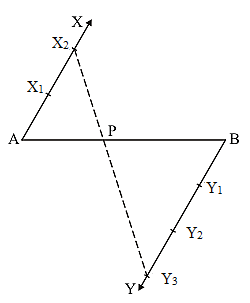Steps of construction:

1. Draw a line segment AB = 12 cm by using a ruler.

2. Through the points A and B draw two parallel line on the opposite side of AB and making the same acute angles with the line segment.

3. Cut 2 equal parts on AX and 3 equal parts on BY such that AX1 = X1X2 and BY= Y1Y2 = Y2Y3.

4. Join X2Y3 which intersects AB at P

Hence,  AP/PB = 2/3.

Justification:

In ΔAX2P and ΔBY3P, we have

∠APX2 = ∠BPY3  [vertically opposite angle]

∠X2AP = ∠Y3BP  [alternate interior angles}

ΔAX2P ΔBY3P  [Because AA similarity]

∴  AP/BP = AX2/BY= 2/3  [From C.P.C.T]

Exercise 11.2 Page No: 11.9

1. Construct a triangle of sides 4 cm, 5 cm and 6 cm and then a triangle similar to it whose sides are (2/3) of the corresponding sides of it.
Solution: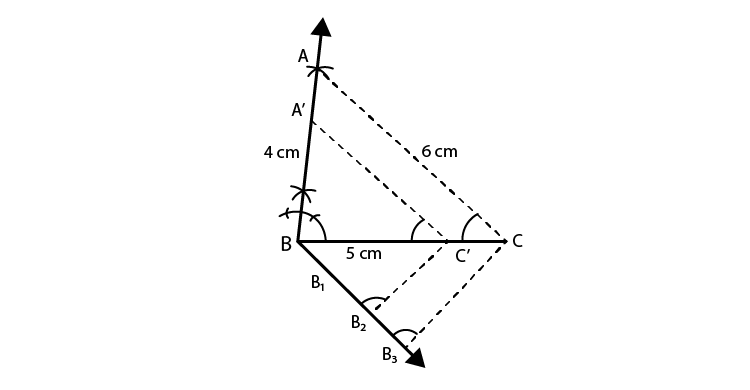Steps of construction:

1. Draw a line segment BC = 5 cm.

2. With centre as B and radius 4 cm and with centre C and radius 6 cm, draw arcs from both points to intersect each other at A.

3. Now, join AB and AC. Then ABC is the triangle.

4. Draw a ray BX making an acute angle with BC and cut off 3 equal parts making BB1 = B1B2= B2B3.

5. Join B3C.

6. Draw B’C’ parallel to B3C and C’A’ parallel to CA.

Then, ΔA’BC’ is the required triangle.

2. Construct a triangle similar to a given ΔABC such that each of its sides is (5/7)th of the corresponding sides of ΔABC. It is given that AB = 5 cm, BC = 7 cm and ∠ABC = 50°.
Solution: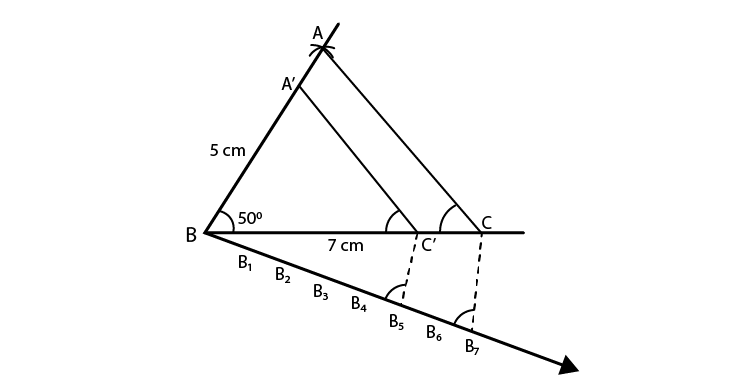Steps of construction:

1. Draw a line segment BC = 7 cm.

2. Draw a ray BX making an angle of 50° and cut off BA = 5 cm.

3. Join AC. Then ABC is the triangle.

4. Draw a ray BY making an acute angle with BC and cut off 7 equal parts making BB1 = B1B2 = B2B3 = B3B4 = B4Bs = B5B6 = B6B7

5. Now, join B7 and C

6. Draw B5C’ parallel to B7C and C’A’ parallel to CA.

Then, ΔA’BC’ is the required triangle.

3. Construct a triangle similar to a given ΔABC such that each of its sides is (2/3)rd of the corresponding sides of ΔABC. It is given that BC = 6 cm, ∠B = 50° and ∠C = 60°.
Solution: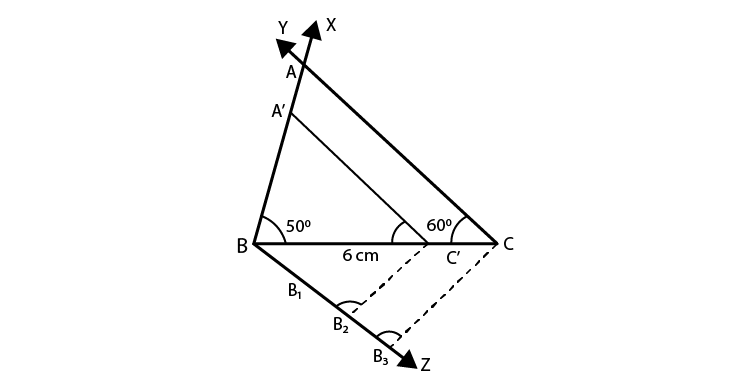Steps of construction:

1. Draw a line segment BC = 6 cm.

2. Draw a ray BX making an angle of 50° and CY making 60° with BC which intersect each other at A. Then, ABC is the triangle.

3. From B, draw another ray BZ making an acute angle below BC and then cut off 3 equal parts making BB1 = B1B2 = B2B2

4. Now, join B3C.

5. From B2, draw B2C’ parallel to B3C and C’A’ parallel to CA.

Then ΔA’BC’ is the required triangle.

4. Draw a ΔABC in which BC = 6 cm, AB = 4 cm and AC = 5 cm. Draw a triangle similar to ΔABC with its sides equal to (3/4)th of the corresponding sides of ΔABC.
Solution: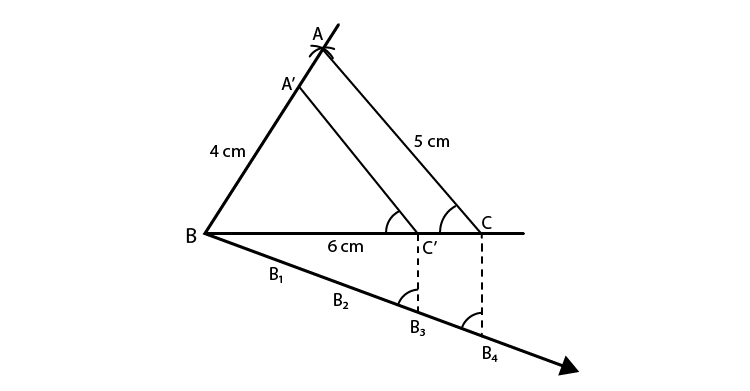Steps of construction:

1. Draw a line segment BC = 6 cm.

2. With centre as B and radius 4 cm and with C as centre and radius 5 cm, draw arcs intersecting each other at A.

3. Join AB and AC. Then, ABC is the triangle.

4. Draw a ray BX making an acute angle with BC and cut off 4 equal parts making BB1 = B1B= B2B3 = B3B4.

5. Join B4 and C’.

6. From B3C draw C3C’ parallel to B4C and from C’, draw C’A’ parallel to CA.

Then ΔA’BC’ is the required triangle.

5. Construct a triangle with sides 5 cm, 6 cm and 7 cm and then another triangle whose sides are (7/5)th of the corresponding sides of the first triangle.
Solution: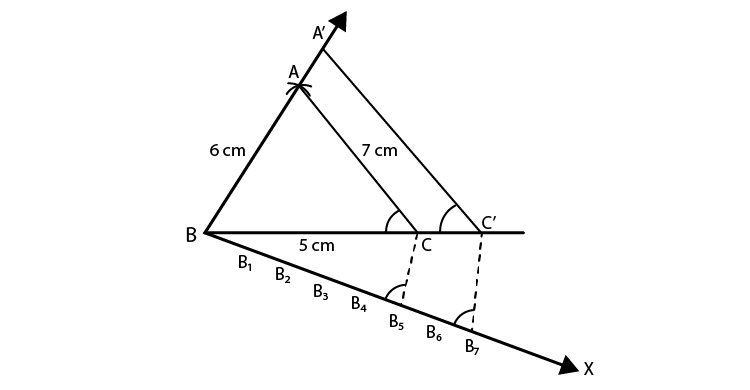Steps of construction:

1. Draw a line segment BC = 5 cm.

2. With B as centre and radius 6 cm and with C as centre and radius 7 cm, draw arcs intersecting each other at A.

3. Now, join AB and AC. Then, ABC is the triangle.

4. Draw a ray BX making an acute angle with BC and cut off 7 equal parts making BB1 = B1B2 = B2B3 = B3B4 = B4B5 = B5B6 = B6B7.

5. Join B5 and C.

6. From B7, draw B7C’ parallel to B5C and C’A’ parallel CA.

Then, ΔA’BC’ is the required triangle.

6. Draw a right triangle ABC in which AC = AB = 4.5 cm and ∠A = 90°. Draw a triangle similar to ΔABC with its sides equal to (5/4)th of the corresponding sides of ΔABC.
Solution: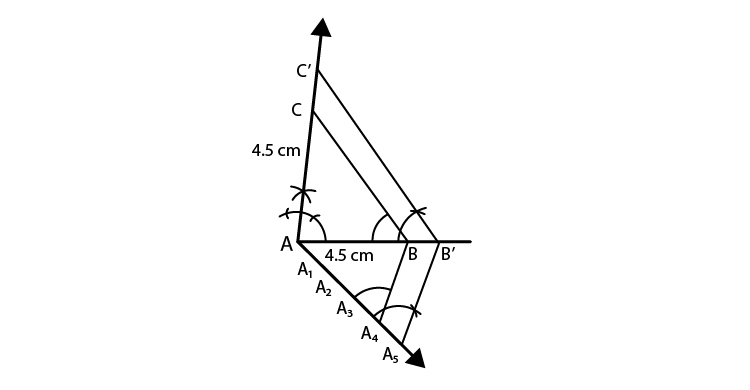Steps of construction:

1. Draw a line segment AB = 4.5 cm.

2. At A, draw a ray AX perpendicular to AB and cut off AC = AB = 4.5 cm.

3. Now, join BC. Then, ABC is the triangle.

4. Draw a ray AY making an acute angle with AB and cut off 5 equal parts making AA1 = A1A2 = A2A3 = A3A4 = A4A5

5. Join A4 and B.

6. From A5, draw A5B’ parallel to  A4B and  B’C’ parallel to BC.

Then, ΔAB’C’ is the required triangle.

7. Draw a right triangle in which the sides (other than hypotenuse) are of lengths 5 cm and 4 cm. Then construct another triangle whose sides are 5/3 times the corresponding sides of the given triangle.
Solution: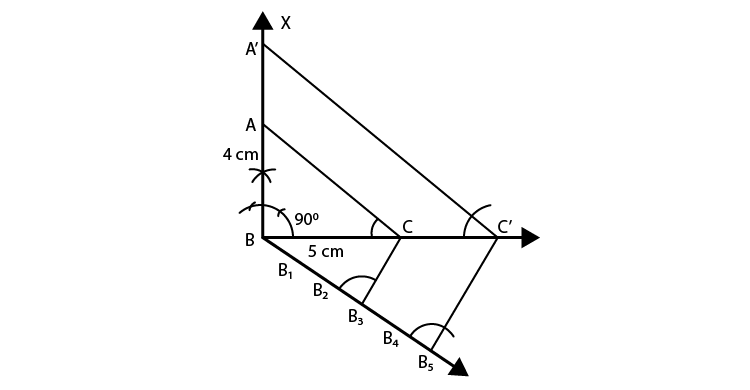Steps of construction:

1. Draw a line segment BC = 5 cm.

2. At B, draw perpendicular BX and cut off BA = 4 cm.

3. Now, join AC. Then, ABC is the triangle

4. Draw a ray BY making an acute angle with BC and cut off 5 equal parts making BB1 = B1B2 = B2B3 = B3B4 = B4B5

5. Join B3 and C.

6. From B5, draw B5C’ parallel to B3C and C’A’ parallel to CA.

Then, ΔA’BC’ is the required triangle.

Exercise 11.3 Page No: 11.17

1. Draw a circle of radius 6 cm. From a point 10 cm away from its centre, construct a pair of tangents to the circle and measure their lengths.

Solution: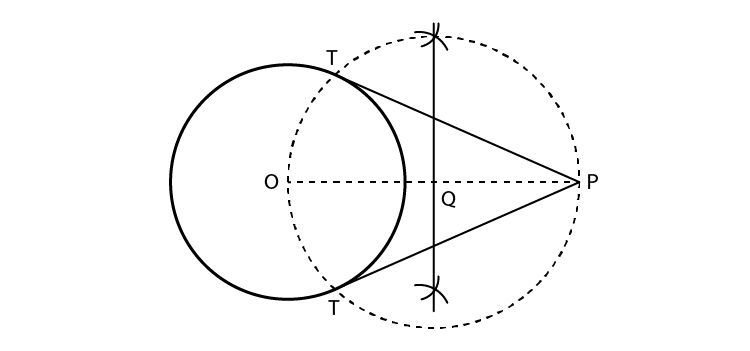Steps of construction:

1. Firstly, we draw a circle with centre O and radius 6 cm.

2. Mark a point P at a distance of OP = 10 cm, and join OP.

3. Draw a right bisector of OP, intersecting OP at Q.

4. Now, taking Q as centre and radius OQ = PQ, draw a circle to intersect the given circle at T and T`.

5. Join PT and P`T` to obtain the required tangents.

Thus, PT and P`T` are the required tangents.

To find the length of the tangents.

We know that OT ⊥ PT and ΔOPT is the right triangle.

Therefore, OT = 6 cm (radius) and PO = 10 cm.

So, in ΔOPT,

PT2  = OP2 − OT2 [By Pythagoras theorem]

= (10)2 − (6)2

= 100 – 36

= 64 PT

= 8 cm

Therefore, the length of tangents 8 cm each.

​2. Draw a circle of radius 3 cm. Take two points P and Q on one of its extended diameter each at a distance of 7 cm from its centre. Draw tangents to the circle from these points P and Q.

Solution: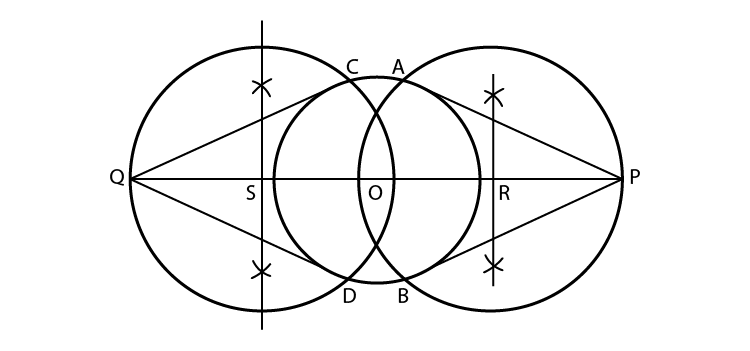Steps of construction:

1. Draw a line segment PQ of 14 cm.

2. Now, mark the midpoint O of PQ.

3. Draw the perpendicular bisectors of PO and OQ which intersects at points R and S on PQ.

4. With centre R and radius RP draw a circle.

5. With centre S and radius, SQ draw a circle.

6. And now, with centre O and radius 3 cm draw another circle which intersects the previous circles at the points A, B, C, and D.

7. Finally, join PA, PB, QC and QD. Thus, PA, PB, QC, and QD are the required tangents.

​3. Draw a line segment AB of length 8 cm. Taking A as centre, draw a circle of radius 4 cm and taking B as the centre, draw another circle of radius 3 cm. Construct tangents to each circle from the centre of the other circle.

Solution: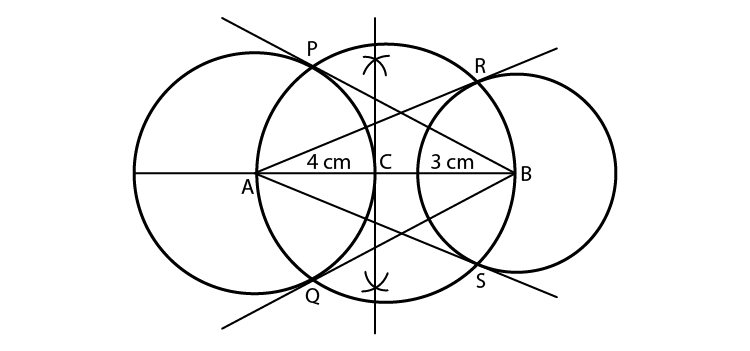Steps of construction:

1. Draw a line segment AB = 8 cm.

2. Draw the perpendicular of AB which intersects it at C.

3. With the centre, C and radius CA draw a circle.

4. Now, with A & B as centres and radii 4 cm and 3 cm respectively, draw two circles which intersects the previous circle at the points P, Q, R and S.

5. Finally, join AR, AS, BP and BQ.

Thus, AR, AS, BP and BQ are the required tangents.